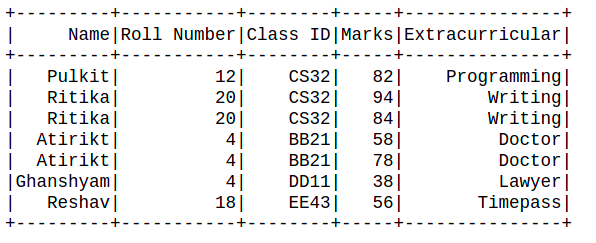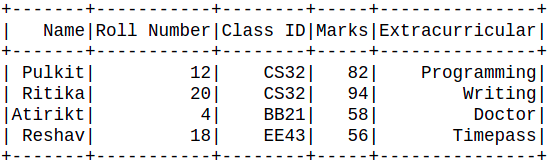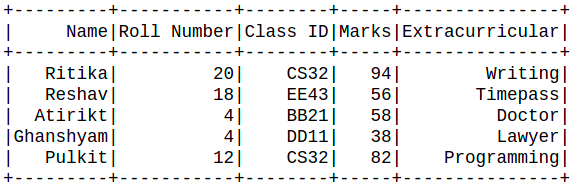# How to drop duplicates and keep one in PySpark dataframe

• Last Updated : 17 Jun, 2021

In this article, we will discuss how to handle duplicate values in a pyspark dataframe. A dataset may contain repeated rows or repeated data points that are not useful for our task. These repeated values in our dataframe are called duplicate values.

To handle duplicate values, we may use a strategy in which we keep the first occurrence of the values and drop the rest.

Attention geek! Strengthen your foundations with the Python Programming Foundation Course and learn the basics.

To begin with, your interview preparations Enhance your Data Structures concepts with the Python DS Course. And to begin with your Machine Learning Journey, join the Machine Learning - Basic Level Course

dropduplicates(): Pyspark dataframe provides dropduplicates() function that is used to drop duplicate occurrences of data inside a dataframe.

Syntax: dataframe_name.dropDuplicates(Column_name)

The function takes Column names as parameters concerning which the duplicate values have to be removed.

Creating Dataframe for demonstration:

## Python3

 `# importing module``import` `pyspark`` ` `# importing sparksession from pyspark.sql module``from` `pyspark.sql ``import` `SparkSession``from` `pyspark.sql.types ``import` `StructType, StructField,``StringType, IntegerType, FloatType`` ` `# Start spark session``spark ``=` `SparkSession.builder.appName(``"Student_Info"``).getOrCreate()`` ` `# Initialize our data``data2 ``=` `[(``"Pulkit"``, ``12``, ``"CS32"``, ``82``, ``"Programming"``),``         ``(``"Ritika"``, ``20``, ``"CS32"``, ``94``, ``"Writing"``),``         ``(``"Ritika"``, ``20``, ``"CS32"``, ``84``, ``"Writing"``),``         ``(``"Atirikt"``, ``4``, ``"BB21"``, ``58``, ``"Doctor"``),``         ``(``"Atirikt"``, ``4``, ``"BB21"``, ``78``, ``"Doctor"``),``         ``(``"Ghanshyam"``, ``4``, ``"DD11"``, ``38``, ``"Lawyer"``),``         ``(``"Reshav"``, ``18``, ``"EE43"``, ``56``, ``"Timepass"``)``         ``]`` ` `# Define schema``schema ``=` `StructType([``    ``StructField(``"Name"``, StringType(), ``True``),``    ``StructField(``"Roll Number"``, IntegerType(), ``True``),``    ``StructField(``"Class ID"``, StringType(), ``True``),``    ``StructField(``"Marks"``, IntegerType(), ``True``),``    ``StructField(``"Extracurricular"``, StringType(), ``True``)``])`` ` `# read the dataframe``df ``=` `spark.createDataFrame(data``=``data2, schema``=``schema)``df.show()`

Output:Examples 1: This example illustrates the working of dropDuplicates() function over a single column parameter. The dataset is custom-built, so we had defined the schema and used spark.createDataFrame() function to create the dataframe.

## Python3

 `# drop duplicates``df.dropDuplicates([``'Roll Number'``]).show()`` ` `# stop Session``spark.stop()`

Output:From the above observation, it is clear that the rows with duplicate Roll Number were removed and only the first occurrence kept in the dataframe.

Example 2: This example illustrates the working of dropDuplicates() function over multiple column parameters. The dataset is custom-built so we had defined the schema and used spark.createDataFrame() function to create the dataframe.

## Python3

 `# drop duplicates``df.dropDuplicates([``'Roll Number'``,``"Name"``]).show()`` ` `# stop the session``spark.stop()`

Output:From the above observation, it is clear that the data points with duplicate Roll Numbers and Names were removed and only the first occurrence kept in the dataframe.

Note: The data having both the parameters as a duplicate was only removed. In the above example, the Column Name of “Ghanshyam” had a Roll Number duplicate value, but the Name was unique, so it was not removed from the dataframe. Thus, the function considers all the parameters not only one of them.

My Personal Notes arrow_drop_up